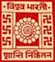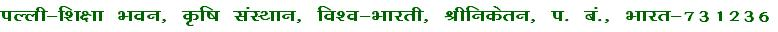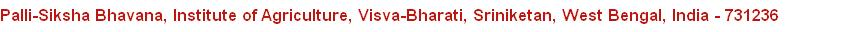Department of Agricultural Statistics e-learning Resources
 Statistical  Calculator
 SPSS Manual
 S.No. Title 1. Statistical Calculator for Descriptive Statistics 2. Statistical Calculator for Correlation 3. Statistical Calculator for Completely Randomized Design (CRD) 4. Statistical Calculator for Randomized Block Design (RBD) 5. Statistical Calculator for Factorial RCB Design 6. Statistical Calculator for Factorial Experiment with Extra Treatments 7. Statistical Calculator for Split Plot Design 8. Statistical Calculator for Pooled Analysis 9. Statistical Calculator for Heritability
 S.No. Title 1. An Overview of SPSS 2. SPSS Practical Manual on Descriptive Statistics 3. SPSS Practical Manual on Correlation Coefficient 4. SPSS Practical Manual on Completely Randomized Design (CRD) 5. SPSS Practical Manual on Randomized Block Design (RBD) 6. SPSS Practical Manual on Latin Square Design (LSD) 7. SPSS Practical Manual on Factorial Design 8. SPSS Practical Manual on Duncan's Multiple Range Test (DMRT) 9. SPSS Practical Manual on Normality Checking of Data 10. SPSS Practical Manual on Principal Component Analysis
 Statistical Tables
 S.No. Title 1. t-table 2. F-table 3. Normal table 4. Chi-square table 5. Random Number Table